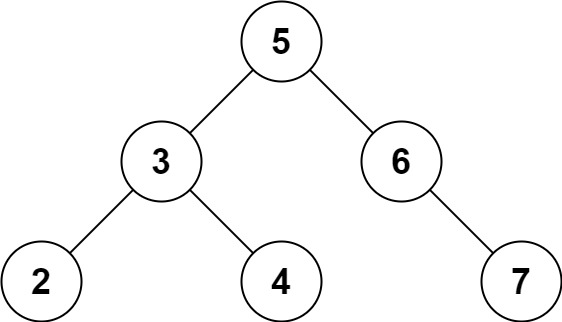# 653. Two Sum IV - Input is a BST

Easy
Given the `root` of a Binary Search Tree and a target number `k`, return `true` if there exist two elements in the BST such that their sum is equal to the given target.
Example 1:Input: root = [5,3,6,2,4,null,7], k = 9
Output:
true
Example 2:Input: root = [5,3,6,2,4,null,7], k = 28
Output:
false
Constraints:
• The number of nodes in the tree is in the range `[1, 104]`.
• `-104 <= Node.val <= 104`
• `root` is guaranteed to be a valid binary search tree.
• `-105 <= k <= 105`

### 解題

Runtime: 29 ms, faster than 81.78%
Memory Usage: 7.1 MB, less than 91.01%
/**
* Definition for a binary tree node.
* type TreeNode struct {
* Val int
* Left *TreeNode
* Right *TreeNode
* }
*/
func findTarget(root *TreeNode, k int) bool {
m := make(map[int]bool)
var helper func(*TreeNode) bool
helper = func(root *TreeNode) bool{
if root == nil {
return false
}
if m[k - root.Val] { return true }
m[root.Val] = true
return helper(root.Left) || helper(root.Right)
}
return helper(root)
}